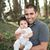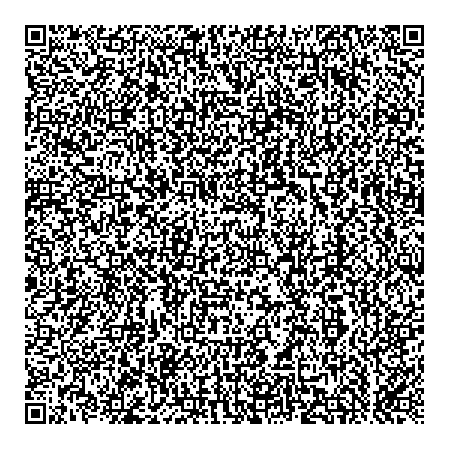# Alok Menghrajani

Previously: security engineer at Square, co-author of HackLang, put the 's' in https at Facebook. Maker of CTFs.

This blog does not use any tracking cookies and does not serve any ads. Enjoy your anonymity; I have no idea who you are, where you came from, and where you are headed to. Let's dream of an Internet from times past.

Have you ever tried to embed a QR code inside a QR code?

Quines are little programs which produce copies of themselves. I figured it would be fun and challenging to try to make a QR code quine.

My QR code contains a piece of javascript which is able to re-generate the same QR code. The javascript is encoded as a data URI. The javascript code reads itself (via location.href) and then generates the same QR code image, ad infinitum.

I started with Jerome Etienne's qrcode.js library, and rewrote the code in a size optimized way. I had to do this because QR codes can only contain a limited number of bytes. My final code is roughly 20x smaller (from ~28,000 to ~1,500 bytes)!Note: some older devices may have trouble scanning large images (due to poor camera quality). I tested this image with an iPhone 5S. When I scan the QR code, I get a blob of data starting with "data:text/html,...". I can then copy-paste this data into Safari and I get the same image back. If your device can't scan the QR code, you can try using an online QR code service.

Here is the data which is embedded in the above image.

You can also trace the steps I took to achieve this.

`data:text/html,<body style=padding:9><canvas id=C><script>function P(r,c,v){I[A*r+c]=v;return!(v&&C.getContext('2d').fillRect(c*3,r*3,3,3))}function S(i,j){for(r=O;r<8;r++)for(c=O;c<8;c++)j+c>O&&j+c<A&&P(i+r,j+c,0<r&&(r<7&&!(c%6))||((c+1)%8&&!(r%6)||1<r&&(r<5&&(1<c&&c<5))))}function N(a,b){if(a[L]<b[L])return a;o=a/b;for(i=0;i<b[L];a[i++]^=z){m=b[i];n=o;for(z=0;m;n>255&&(n^=285))m&1&&(z^=n),n<<=1,m>>=1;}a.shift();return N(a,b)}C.height=C.width=(A=133)*3;I=[];X=O=-1;L='length';S(0,0);S(A-7,0);S(i=0,A-7);for(B=[6,30,54,78,102,126];i<6;i++)for(j=0;j<6;j++)if(!I[A*B[i]+B[j]])for(r=-2;r<3;r++)for(c=-2;c<3;c++)P(B[i]+r,B[j]+c,r&&!(r%2)||c&&!(c%2)||!r&&!c);for(i=8;i<A-8;P(6,i++,j))j=!(i%2),P(i,6,j);for(i=0;i<15;i++)j=29427>>i&1,i<6&&P(i,8,j)||i<8&&P(i+1,8,j)||P(118+i,8,j),i<8&&P(8,A-i-1,j)||i<9&&P(8,15-i,j)||P(8,14-i,j);P(A-8,8,1);for(i=0;i<18;P(i%3+A-11,i++/3|0,k))k=119615>>i&1,P(i/3|0,i%3+A-11,k);for(D=[4,i=0,6,0,6];i<1542;D=D.concat([j>>4,j&15]))j=unescape(location.href).charCodeAt(i++);D.push(r=0);for(E=3262;D[L]<E;D=D.concat([1,1]))D=D.concat([14,12]);F=[];for(Z=k=r=0;r<14;r++){i=116+(r>6);l=[];for(j=0;j<i;F[r+j*14-(j>115?7:0)]=l[j++])l[j]=(D[k++]<<4)|D[k++];l=l.concat(new Array(30));for(j=0;j<30;j++)F[r+j*14+1631]=N(l,[1,212,246,77,73,195,192,75,98,5,70,103,177,22,217,138,51,181,246,72,25,18,46,228,74,216,195,11,106,130,150])[j]}Y=7;for(x=i=A-1;i>0;i-=2){for(i==6&&i--;x>O&&x<A;x+=X)for(j=0;j<2;j++)if(I[A*x+i-j]==B)k=Z<F[L]&&F[Z]>>Y&1,P(x,i-j,x%2?k:!k),--Y<0&&(Z++,Y=7);x-=X;X=-X}console.log('-- Alok')</script>`